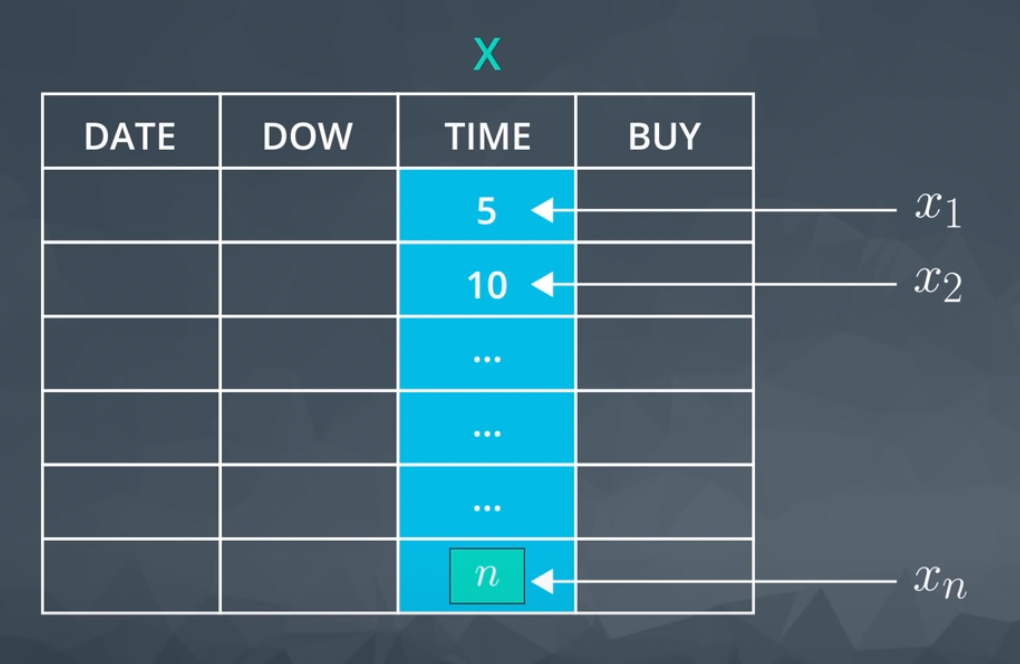# What is data?

• Data: distinct pieces of information

# Data Types

Quantitative data takes on numeric values that allow us to perform mathematical operations (like the number of dogs).We can think of quantitative data as being either continuous or discrete.

• Continuous data can be split into smaller and smaller units, and still a smaller unit exists. An example of this is the age.
• Discrete data only takes on countable values. The number of dogs.

Categorical are used to label a group or set of items (like dog breeds — Collies, Labs, Poodles, etc.). We can divide categorical data further into two types: Ordinal and Nominal.

• Categorical Ordinal data take on a ranked ordering (like a ranked interaction on a scale from `Very Poor` to `Very Good` with the dogs).
• Categorical Nominal data do not have an order or ranking (like the breeds of the dog).

# Measures of Centre (3M’s: Mean, Median, Mode)

## Mean

• Def: Sum of all values divided by the count of values or the expected value in the mathematics.
• 有離群值不適用、且會把答案變成小數點。

## Median

• Def: The middle value of a data set，取決於是偶數個還是奇數個的資料集
• Median of odd value(Direct middle): n = 7 > 7 + 1 / 2 = 4 > 第四個值是中位數。
• Median of even value(The average of the two value in the middle): n = 8，8 / 2 = 4 > 第四個與第五個值去做平均就是中位數。

## Mode

• Def: 最常見的那個值
• 可能沒有，也可能有很多個。

# What is Notation?

• Def: Common math language used to communicate.
• Think of notation as a universal language used by academic and industry professionals to convey mathematical ideas.
• 很重要。能夠簡單化你想傳達的東西，讓你能夠閱讀許多演算法相關的文件 .etc

# Random Variables

• Each column in a spreadsheet commonly holds a specific variable, while each row is commonly called an instance or individual.
• Def: Placeholder for the possible values of some process. Notation=X
• X is an entire set of possible values.

# Capital vs. LowerR.V. — Capital letter, Observed values — Lowercase letters

# Measures of Spread — How far are points from one another

• Range
• Interquartile Range (IQR)
• Standard Deviation
• Variance

• 直方圖。

# 5 Number Summary

• Def: Gives values for calculating the RANGE and IQR
• 怎麼找呢？先排序，就可以找到最大值、最小值跟中位數(i.e. Q2)。
• Q1跟 Q3 則是 Q2 兩側的中位數。
• Box plot — Useful for quickly comparing the spread of two data sets. For datasets that are not symmetric, the five number summary and a corresponding box plot are a great way to get started with understanding the spread of your data. Although I still prefer a histogram in most cases, box plots can be easier to compare two or more groups.

# Standard Deviation and Variance

• It is defined as the average distance of each observation from the mean.

## Important Final Points

1. 變異數通常是來比較兩個群體的分散趨勢。有較高的變異數的資料集會比較低的更為分散。離群值會增加變異數的值。
2. 當比較兩個資料集的分散趨勢時，一定要同單位。
3. 當資料與錢或者是經濟學有相關，高變異數或標準差通常與高風險有關。
4. 實務上，標準差比變異數更常使用，因為他是原單位（變異數則是單位的平方）

# Outliers

• Def: Data points that fall very far from the rest of the values in our dataset

## Common Techniques

When outliers are present we should consider the following points.

1. Noting they exist and the impact on summary statistics.

2. If typo — remove or fix

3. Understanding why they exist, and the impact on questions we are trying to answer about our data.

4. Reporting the 5 number summary values is often a better indication than measures like the mean and standard deviation when we have outliers.

5. Be careful in reporting. Know how to ask the right questions.

1. Plot your data to identify if you have outliers.

2. Handle outliers accordingly via the methods above.

3. If no outliers and your data follow a normal distribution — use the mean and standard deviation to describe your dataset, and report that the data are normally distributed.

## Side note

If you aren’t sure if your data are normally distributed, there are plots called normal quantile plots and statistical methods like the Kolmogorov-Smirnov test that are aimed to help you understand whether or not your data are normally distributed. Implementing this test is beyond the scope of this class, but can be used as a fun fact.

4. If you have skewed data or outliers, use the five number summary to summarize your data and report the outliers.

# 敘述統計與推論統計

## Descriptive Statistics

`Descriptive statistics` is about describing our collected data.

## Inferential Statistics

`Inferential Statistics` is about using our collected data to draw conclusions to a larger population.

We looked at specific examples that allowed us to identify the

1. Population — our entire group of interest.
2. Parameter — numeric summary about a population
3. Sample — subset of the population
4. Statistic numeric summary about a sample

--

--